Computer Vision for the Web3.8 (4 reviews total)
• \$5/mo for 5 months Subscribe Access now
• \$23.99 eBook

• Constantly updated with 100+ new titles each month
• Breadth and depth in over 1,000+ technologies

JavaScript is a dynamic and prototype-based programming language supported by every browser today. JavaScript libraries boast outstanding functionalities that enable you to furnish your own Computer Vision projects, making it easier to develop JavaScript–based applications, especially for web-centric technologies. It makes the implementation of Computer Vision algorithms easier as it supports scheme-based functional programming.

This book will give you an insight into controlling your applications with gestures and head motion and readying them for the web. Packed with real-world tasks, it begins with a walkthrough of the basic concepts of Computer Vision that the JavaScript world offers us, and you’ll implement various powerful algorithms in your own online application. Then, we move on to a comprehensive analysis of JavaScript functions and their applications. Furthermore, the book will show you how to implement filters and image segmentation, and use tracking.js and jsfeat libraries to convert your browser into Photoshop. Subjects such as object and custom detection, feature extraction, and object matching are covered to help you find an object in a photo. You will see how a complex object such as a face can be recognized by a browser as you move toward the end of the book. Finally, you will focus on algorithms to create a human interface.

By the end of this book, you will be familiarized with the application of complex Computer Vision algorithms to develop your own applications, without spending much time learning sophisticated theory.

Publication date:
October 2015
Publisher
Packt
Pages
116
ISBN
9781785886171

Chapter 1. Math Never Was So Simple!

Computer Vision is all about math. When you need to create your own algorithm or implement something, you address a math topic. You should know how it works on the inside because without digging into the basics, it is hard to do anything. But you are not alone! Many smart people have created several useful libraries to simplify your job. One of those libraries is JSFeat (http://inspirit.github.io/jsfeat/), which has a realization of different math methods. Here, we will discuss fundamental elements of the library such as data structures, especially matrices, and simple math algorithms.

We will cover the following topics:

• Installation and core structure representation of JSFeat

• What is inside an image? All about matrices

• Useful functions and where to use them

Installation and core structure representation of JSFeat

JSFeat is a powerful tool to implement something new. To start using it, we need to initialize the project. It is relatively simple; if you have any experience with JavaScript, then it will not cause any trouble for you. The library itself contains various Computer Vision algorithms and it will be a good starting point for anyone who wants a flexible Computer Vision framework. First, you will learn how to install it and see a basic example of what you can do with the library.

Initializing the project

First of all, you need to download the JSFeat library and add it to your webpage. It is simple and it looks similar to this:

<!doctype html>
<html>
<meta charset="utf-8">
<title>chapter1</title>
<script src="js/jsfeat.js"></script>
<body></body></html>

As you can see, we just added a JavaScript library here without any additional actions. We do not need any particular software, since JavaScript is fast enough for many Computer Vision tasks.

The core data structure for the JSFeat library is a matrix. We will cover more topics about matrices in the next section, but to check whether everything works correctly, let's try to create an example.

Add the following code to a <script/> tag:

var matrix = new jsfeat.matrix_t(3, 3, jsfeat.U8_t | jsfeat.C1_t);
matrix.data = 1;
matrix.data = 2;
matrix.data = 1;
for (var i = 0; i < matrix.rows; ++i) {
var start = i * matrix.cols;
console.log(matrix.data.subarray(start, start + matrix.cols));
}

You will see the following in your console:

[0, 1, 0]
[0, 0, 2]
[0, 1, 0]

In the preceding code, we create a new matrix with the dimensions of 3 x 3 and an unsigned byte type with one channel. Next, we set a few elements into it and log the content of the matrix into the console row by row. The matrix data is presented as a one-dimensional array. Remember this, we will clarify it in the next section.

Finally, you did it! You have successfully added the JSFeat Computer Vision library to your first project. Now, we will discuss what a matrix actually is.

Understanding a digital image

It is likely that you already know that an image consists of pixels, which is a big step in understanding image processing. You already saw in the previous topics that a matrix is just a one-dimensional array. However, it represents two-dimensional array and its elements are presented in a row-major order layout. It is more efficient in terms of speed and memory to create a matrix in such a way. Our images are two dimensional too! Each pixel reflects the value of an array element. Consequently, it is obvious that a matrix is the best structure for image representation. Here, we will see how to work with a matrix and how to apply matrix conversion operations on an image.

The JSFeat library uses its own data structure for matrices. First, we load an image using regular HTML and JavaScript operations. We then place a canvas on our webpage:

<canvas id="initCanvas"></canvas>

Then we need to place an image here. We do this with just a few lines of code:

var canvas = document.getElementById('initCanvas'),
context = canvas.getContext('2d'),
image = new Image();
image.src = 'path/to/image.jpg';

var cols = image.width;
var rows = image.height;
canvas.width = cols;
canvas.height = rows;
context.drawImage(image, 0, 0, image.width, image.height);
};

This is just a common way of displaying an image on a canvas. We define the image source path, and when the image is loaded, we set the canvas dimensions to those of an image and draw the image itself. Let's move on. Loading a canvas' content into a matrix is a bit tricky. Why is that? We need to use a jsfeat.data_t method, which is a data structure that holds a binary representation of an array. Anyway, since it is just a wrapper for the JavaScript ArrayBuffer, it should not be a problem:

var imageData = context.getImageData(0, 0, cols, rows);
var dataBuffer = new jsfeat.data_t(cols * rows, imageData.data.buffer);
var mat = new jsfeat.matrix_t(cols, rows, jsfeat.U8_t | jsfeat.C4_t, dataBuffer);

Here, we create a matrix as we did earlier, but in addition to that we add a new parameter, matrix buffer, which holds all the necessary data.

Probably, you already noticed that the third parameter for the matrix construction looks strange. It sets the type of matrix. Matrices have two properties:

• The first part represents the type of data in the matrix. In our example, it is U8_t; it states that we use unsigned byte array. Usually, an image uses 0-255 range for a color representation, that is why we need bytes here.

• Remember that an image consists of 3 main channels (red, green, and blue) and an alpha channel. The second part of the parameter shows the number of channels we use for the matrix. If there is only one channel, then it is a grayscale image.

How do we convert a colored image into a grayscale image? For the answer, we must move to the next section.

Basic matrix operations

Working with matrices is not easy. Who are we to fear the difficulties? With the help of this section, you will learn how to combine different matrices to produce interesting results.

Basic operations are really useful when you need to implement something new. Usually, Computer Vision uses grayscale images to work with them, since most Computer Vision algorithms do not need color information to track the object. As you may already know, Computer Vision mostly relies on the shape and intensity information to produce the results. In the following code, we will see how to convert a color matrix into a grayscale (one channel) matrix:

var gray = new jsfeat.matrix_t(mat.cols, mat.rows, jsfeat.U8_t | jsfeat.C1_t);
jsfeat.imgproc.grayscale(mat.data, mat.cols, mat.rows, gray);

Just a few lines of code! First, we create an object, which will hold our grayscale image. Next, we apply the JSFeat function to that image. You may also define matrix boundaries for conversion, if you want. Here is the result of the conversion: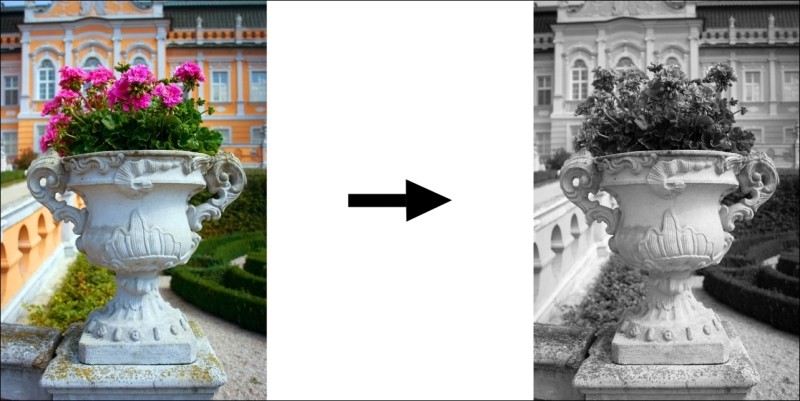For this type of operation, you do not actually need to load a color image into the matrix; instead of mat.data, you can use imageData.data from the context—it's up to you.

To see how to display a matrix, refer to the Matrix displaying section.

One of the useful operations in Computer Vision is a matrix transpose, which basically just rotates a matrix by 90 degrees counter-clockwise. You need to keep in mind that the rows and columns of the original matrix are reflected during this operation:

var transposed = new jsfeat.matrix_t(mat.rows, mat.cols, mat.type | mat.channel);
jsfeat.matmath.transpose(transposed, mat);

Tip

You can download the example code files for all Packt books you have purchased from your account at http://www.packtpub.com. If you purchased this book elsewhere, you can visit http://www.packtpub.com/support and register to have the files e-mailed directly to you. Download link for the book: https://github.com/foat/computer-vision-for-the-web.

Again, we need to predefine the resulting matrix, and only then we can apply the transpose operation: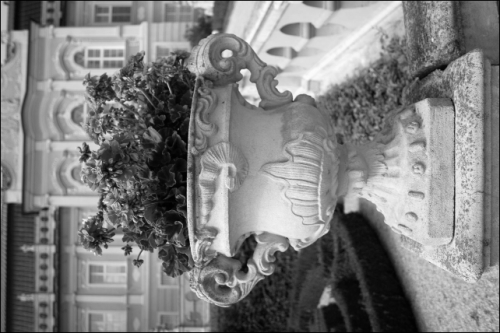Another operation that can be helpful is a matrix multiplication. Since it is hard to see the result on an image, we will fill matrices manually. The following code works by the formula C = A * B, the number of rows of the first matrix must be equal to the number of columns of the second matrix, e.g. MxN and NxK, those are dimensions for the first and the second matrices accordingly:

var A = new jsfeat.matrix_t(2, 3, jsfeat.S32_t | jsfeat.C1_t);
var B = new jsfeat.matrix_t(3, 2, jsfeat.S32_t | jsfeat.C1_t);
var C = new jsfeat.matrix_t(3, 3, jsfeat.S32_t | jsfeat.C1_t);
for (var i = 0; i < A.data.length; ++i) {
A.data[i] = i + 1;
B.data[i] = B.data.length / 2 - i;
}
jsfeat.matmath.multiply(C, A, B);

Here, the M = K = 3 and N = 2. Keep in mind that during the matrix creation, we place columns as a first parameter, and only as the second do we place rows. We populate matrices with dummy values and call the multiply function. After displaying the result in the console, you will see this:

[1, 2] [3,  2,  1] [ 3,  0, -3]
[3, 4] [0, -1, -2] [-3,  9,  2]
[5, 6]             [ 2, -5, 15]

Here the first column is matrix A, the second – matrix B and the third column is the result matrix of C.

JSFeat also provides such functions for matrix multiplication as multiply_ABt, multiply_AAt, and so on, where t means transposed. Use these functions when you do not want to write additional lines of code for the transpose method. In addition to this, there are matrix operations for 3 x 3 matrices, which are faster and optimized for this dimension. Besides, they are useful when, for example, you need to work with coordinates.

In the two-dimensional world, we use only x and y for coordinates. However, for more complex algorithms, when we need to define a point of intersection between two parallel lines, we need to add z (third) coordinate to a point, this system of coordinates is called homogeneous coordinates. They are especially helpful when you need to project a three-dimensional object onto a two-dimensional space.

Going deeper

Consider find features on an image, these features are usually used for object detection. There are many algorithms for this but you need a robust approach, which has to work with different object sizes. Moreover, you may need to reduce the redundancy of an image or search something the size of which you are unsure of. In that case, you need a set of images. The solution to this is a pyramid of an image. An image pyramid is a collection of several images, which are downsampled from the original.

The code for creating an image pyramid will look like this:

var levels = 4, start_width = mat.cols, start_height = mat.rows,
data_type = jsfeat.U8_t | jsfeat.C1_t;
var pyramid = new jsfeat.pyramid_t(levels);
pyramid.allocate(start_width, start_height, data_type);
pyramid.build(mat);

First, we define the number of levels for the pyramid; here, we set it to 4. In JSFeat, the first level is skipped by default, since it is the original image. Next, we define the starting dimensions and output types. Then, we allocate space for the pyramid levels and build the pyramid itself. A pyramid is generally downsampled by a factor of 2: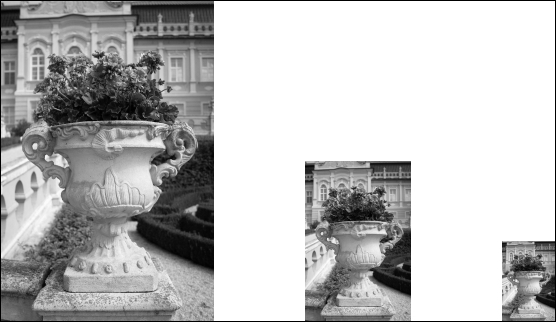JSFeat pyramid is just an array of matrices, it shows different pyramid layers starting from the original image and ending with the smallest image in the pyramid.

Matrix displaying

What we did not discuss in the previous section is how to display output matrices. It is done in different ways for grayscale and colored images. Here is the code for displaying matrices for a colored image:

var data = new Uint8ClampedArray(matColour.data);
var imageData = new ImageData(data, matColour.cols, matColour.rows);
context.putImageData(imageData, 0, 0);

We just need to cast the matrix data to the appropriate format and put the resulting ImageData function into the context. It is harder to do so for a grayscale image:

var imageData = new ImageData(mat.cols, mat.rows);
var data = new Uint32Array(imageData.data.buffer);
var alpha = (0xff << 24);
var i = mat.cols * mat.rows, pix = 0;
while (--i >= 0) {
pix = mat.data[i];
data[i] = alpha | (pix << 16) | (pix << 8) | pix;
}

This is a binary data representation. We populate the ImageData function with the alpha channel, which is constant for all pixels as well as for red, green, and blue channels. For a gray image, they have the same value, which is set as the pix variable. Finally, we need to put the ImageData function into the context as we did in the previous example.

Useful functions and where to use them

There are many functions that are needed in Computer Vision. Some of them are simple, such as sorting, while others are more complex. Here, we will discuss how to use them with the JSFeat library and see several Computer Vision applications.

Sorting using JSFeat

Sort algorithms are always helpful in any application. JSFeat provides an excellent way to sort a matrix. In addition to just sorting an array, it can even sort just part of the data. Let's see how we can do that:

1. First, we need to define a compare function, which is as follows:

var compareFunc = function (a, b) {
return a < b;
};
2. Next, we do the sorting:

var length = mat.data.length;
jsfeat.math.qsort(mat.data, length / 3 * 2, length - 1, compareFunc);

The first parameter defines an array for sorting, the second and third are the starting index and the ending index, respectively. The final parameter defines the comparison function. You will see the following image: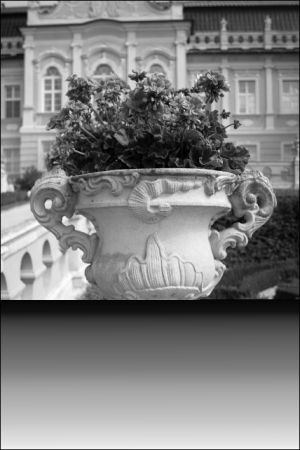As we can see, the lower portion part of the image was sorted, looks good!

You will probably need a median function, which returns the number that separates the higher part of the data from the lower part. To understand this better, we need to see some examples:

var arr1 = [2, 3, 1, 8, 5];
var arr2 = [4, 6, 2, 9, -1, 6];
var median1 = jsfeat.math.median(arr1, 0, arr1.length - 1);
var median2 = jsfeat.math.median(arr2, 0, arr2.length - 1);

For the first array, the result is 3. It is simple. For the sorted array, number 3 just separates 1, 2 from 5, 8. What we do see for the second array, is the result of 4. Actually, different median algorithms may return different results; for the presented algorithm, JSFeat picks one of the array elements to return the result. In contrast, many approaches will return 5 in that case, since 5 represents the mean of two middle values (4, 6). Taking that into account, be careful and see how the algorithm is implemented.

Linear algebra

Who wants to solve a system of linear equations? No one? Don't worry, it can be done very easily.

First, let's define a simple linear system. To start with, we define the linear system as Ax = B, where we know A and B matrices and need to find x:

var bufA = [9, 6, -3, 2, -2, 4, -2, 1, -2],
bufB = [6, -4, 0];

var A = new jsfeat.matrix_t(3, 3, jsfeat.F32_t | jsfeat.C1_t, new jsfeat.data_t(bufA.length, bufA));
var B = new jsfeat.matrix_t(3, 1, jsfeat.F32_t | jsfeat.C1_t, new jsfeat.data_t(bufB.length, bufB));

jsfeat.linalg.lu_solve(A, B);

JSFeat places the result into the B matrix, so be careful if you want to use B somewhere else or you will loose your data. The result will look like this:

[2.000..., -4.000..., -4.000..]

Since the algorithm works with floats, we cannot get the exact values but after applying a round operation, everything will look fine:

[2, -4, -4]

In addition to this, you can use the svd_solve function. In that case, you will need to define an X matrix as well:

jsfeat.linalg.svd_solve(A, X, B);

A perspective example

Let us show you a more catchy illustration. Suppose you have an image that is distorted by perspective or you want to rectify an object plane, for example, a building wall. Here's an example: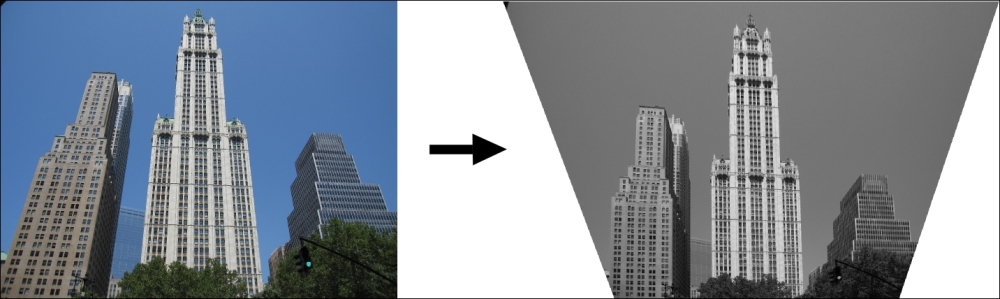Looks good, doesn't it? How do we do that? Let's look at the code:

var imgRectified = new jsfeat.matrix_t(mat.cols, mat.rows, jsfeat.U8_t | jsfeat.C1_t);
var transform = new jsfeat.matrix_t(3, 3, jsfeat.F32_t | jsfeat.C1_t);

jsfeat.math.perspective_4point_transform(transform,
0, 0, 0, 0, // first pair x1_src, y1_src, x1_dst, y1_dst
640, 0, 640, 0, // x2_src, y2_src, x2_dst, y2_dst and so on.
640, 480, 640, 480,
0, 480, 180, 480);
jsfeat.matmath.invert_3x3(transform, transform);
jsfeat.imgproc.warp_perspective(mat, imgRectified, transform, 255);

Primarily, as we did earlier, we define a result matrix object. Next, we assign a matrix for image perspective transformation. We calculate it based on four pairs of corresponding points. For example, the last, that is the fourth point of the original image, which is [0, 480], should be projected to the point of [180, 480] on the rectified image. Here, the first coordinate refers to X and the second to Y. Then, we invert the transform matrix to be able to apply it to the original image—mat variable. We pick the background color as white (255 for an unsigned byte). As a result, we get a nice image without any perspective distortion.

Summary

In this chapter, we saw many useful Computer Vision applications. Every time you want to implement something new, you need to start from the beginning. Fortunately, there are many libraries that can help you with your investigation. Here, we mainly covered the JSFeat library, since it provides basic methods for Computer Vision applications. We discussed how and when to apply the core of this library. Nevertheless, this is just a starting point, and if you want to see more exciting math topics and dig into the Computer Vision logic, we strongly encourage you to go through the next chapters of this book. See you there!